The Repetition Control Structure

The repetition control structure is also known as the looping or iteration control structure.  Looping is the process of repeatedly executing one or more steps of an algorithm or program; it is essential in programming, as most programs perform repetitious tasks.

Every loop consists of the following three parts:

(1)        The loop termination decision - determines when (under what condition) the loop will be terminated (stopped).  It is essential that some provision in the program be made for the loop to stop; otherwise, the computer would continue to execute the loop indefinitely - a loop that doesn't stop is called an endless loop or infinite loop; when a program gets stuck in an endless loop, programmers say that the program is "looping".

(2)        The body of the loop - the step or steps of the algorithm that are repeated within the loop.  This may be only one action, or it may include almost all of the program statements.  Important note: some action must occur within the body of the loop that will affect the loop termination decision at some point.

(3)        Transfer back to the beginning of the loop - returns control to the top of the loop so that a new repetition (iteration) can begin.

There are two basic types of loops: the pre-test loop and the post-test loop.

The Pre-Test Loop

The pre-test loop can be implemented in VB with a "Do" statement followed by either the keyword "While" or "Until".  "Do While" means execute the statements in the body of the loop While a certain condition is true.  "Do Until" means execute the statements in the body of the loop Until a certain condition is true.  While and Until are opposites of each other.  In other words, doing something Until a condition is TRUE is the same as doing something While a condition is FALSE.  For example, Do While X <= 10 is the same as Do Until X > 10.

Both the "While" and the "Until" versions of the pre-test loop are flowcharted below.  The only difference between the two is the placement of the "True" and "False" paths extending from the decision diamond.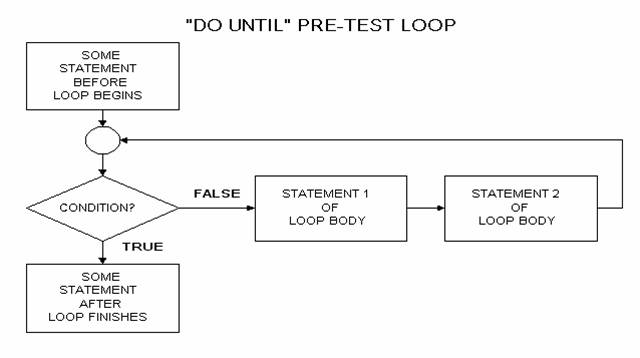With  a pre-test loop, since the "loop termination decision" is tested at the top of the loop, it is possible that the statements in the body of the loop will never be executed.

### The Do While (Do Until) Loop

The general format for a pre-test loop in VB is:

Do {While | Until} <condition>

<list of statements>

Loop

The Do While form of this statement works as follows: First, the condition is evaluated.  If the condition is TRUE, the list of statements is executed and the Loop statement sends control back to the Do While statement, where the condition is re-evaluated. If the condition is FALSE, program control branches to the statement following the Loop statement.

The Do Until form of this statement works as follows: First, the condition is evaluated.  If the condition is FALSE, the list of statements is executed and the Loop statement sends control back to the Do Until statement, where the condition is re-evaluated. If the condition is TRUE, program control branches to the statement following the Loop statement.

A typical application for the pre-test loop is the "input loop".  The input loop works as follows:

(1)  A "priming", or initial Input is performed outside the loop.  It obtains the initial value for the variable that will control the loop termination decision (also called the "loop control variable"; it will determine whether or not to enter the loop for the first time).

(2)  The loop begins with a Do While or Do Until statement.  The condition following one of these compares the loop control variable to a value that will signify the end of the loop.  Such values are called sentinel or terminating values.

(3)  The body of the loop contains the statements that will process each set of input values.  The last statement in the body of the loop should be an Input to obtain another value for the loop control variable.

To demonstrate the coding of an input loop, set up a "Try It" project, and place the following code in the cmdTryIt_Click event:

Private Sub cmdTryIt_Click()

Dim dblNumber   As Double

Dim dblTotal    As Double

dblNumber = Val(InputBox("Enter a number (zero to quit):", "Input Loop"))

Do Until dblNumber = 0

dblTotal = dblTotal + dblNumber

dblNumber = Val(InputBox("Enter a number (zero to quit):", "Input Loop"))

Loop

Print "The total input was: "; Format\$(dblTotal, "Standard")

End Sub

Run the project and click the "Try It" button. Will be prompted repeatedly to enter a number; when you enter zero the prompting will stop and the total of all the numbers that you input will be displayed on the form.

Screen shot of the input box: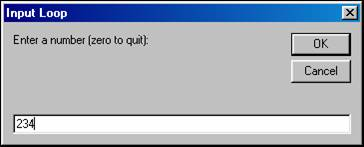Screen shot of the form showing the total:Note that in the sample program the "Until" form of the pre-test loop was used:

Do Until dblNumber = 0

The equivalent "While" form would be:

Do While dblNumber <> 0

The Until form was chosen because the use of "positive" logic in conditions is preferred over the use of "not" logic (i.e., it was preferable to use the "equal" relational operator as opposed to the "not equal" operator).  Positive logic is more readable and less confusing than "not" logic, and should be used whenever possible.

Other VB facilities for pre-test loops are the While/Wend loop and the For/Next loop.

### The While/Wend Loop

The While/Wend loop is an older statement pair from previous versions of BASIC and was included in VB for compatibility.  The format is:

While <condition>

<list of statements>

Wend

The following two code segments produce the exact same results:

 intNum = Val(InputBox("Enter a number")) Do While intNum > 0     intNumSq = intNum ^ 2 Print intNum; "squared is"; intNumSq     intNum = Val(InputBox("Enter a number")) Loop intNum = Val(InputBox("Enter a number")) While intNum > 0     intNumSq = intNum ^ 2     Print intNum; "squared is"; intNumSq     intNum = Val(InputBox("Enter a number")) Wend

There is no "Until" equivalent of "While/Wend".

### The For/Next Loop

The For/Next loop uses a built-in counting procedure to repeat a series of instructions a specific number of times.  Let us compare counting with Do/Loop vs. counting with For/Next.  With Do While, you could set up a loop to print the numbers from 1 to 5 as follows:

intCounter = 1

Do While intCounter <= 5

Print intCounter

intCounter = intCounter + 1

Loop

With a For/Next loop, the above can be shortened to:

For intCounter = 1 TO 5

Print intCounter

Next

The general format of the For/Next loop is as follows:

For <loop control variable> = <initial value> TO <stop value> [Step increment value]

<list of statements>

Next [loop control variable]

When the loop begins, the loop control variable (LCV) will be initialized with the value of the initial value.  Subsequently, a test will be made to see if the LCV exceeds the stop value.  If the LCV exceeds the stop value, the loop will end, and control will pass to whatever statement follows the Next statement.

If the LCV is less than or equal to the stop value, the body of the loop will be executed, and the Next statement automatically increments the LCV by the value specified after the keyword Step (if the Step clause is omitted, the increment value is assumed to be 1).  The Next statement also causes a transfer of control back to the For statement to begin another repetition of the loop.  Note from the general format that the LCV may optionally be specified after the keyword Next, however, programming literature states that it is more efficient to leave it off.

Other notes regarding the For/Next loop:

(1)        The Step value need not be 1.  The statements

For I = 1 TO 10 Step 2

Print I

Next

would produce the following output:

1

3

5

7

9

(2)        The Step value can be negative, in which case the loop executes until the LCV is less than the stop value - therefore, the initial value should be greater than the stop value.  The statements

For X = 3 TO 1 Step -1

Print X

Next

would produce the following output:

3

2

1

(3)        Assuming that the Step value is positive (or the default of 1 is used), if the stop value is less than the initial value when the loop begins, the loop will never execute.  For example, the statements in the body of this loop will never execute:

For X = 5 to 1

(whatever)

Next

Likewise, if the Step value is negative, if the stop value is greater than the initial value when the loop begins, the loop will never execute.  For example, the statements in the body of this loop will never execute:

For X = 1 TO 5 Step -1

(whatever)

Next

The For/Next loop can be flowcharted the same way as its “Do While” counterpart.  A few paragraphs ago, we said that these two pieces of code are equivalent:

 intCounter = 1       Do While intCounter <= 5           Print intCounter           intCounter = intCounter + 1       Loop For intCounter = 1 TO 5           Print intCounter       Next

Therefore, the flowchart would be:To demonstrate the coding of a For/Next loop, we can write a simple program that computes and displays the factorial for a positive integer input by the user.  In algebra, n! (read as "n factorial") is the product of all whole numbers from 1 up to that number.  For example, 5! = 1 x 2 x 3 x 4 x 5 = 120.

Still sticking with our "Try It" format, set up a "Try It" project, and place the following code in the cmdTryIt_Click event:

Private Sub cmdTryIt_Click()

Dim lngFactorial    As Long

Dim intInputNbr     As Integer

Dim intLoopCtr      As Integer

intInputNbr = Val(InputBox("Enter a number:", "For/Next Loop"))

lngFactorial = 1

For intLoopCtr = 1 To intInputNbr

lngFactorial = lngFactorial * intLoopCtr

Next

Print CStr(intInputNbr); "! = "; lngFactorial

End Sub

Run the project and click the Try It button.  Input a number and its factorial will be printed on the form.

Screen shot of the input box: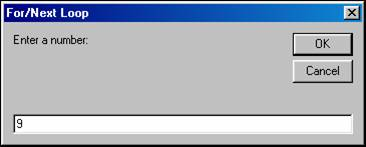Screen shot of the form showing the factorial: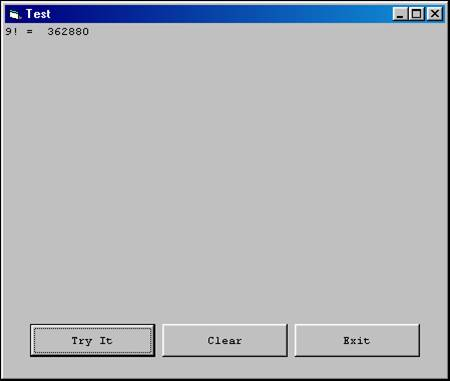Note: This example can handle a maximum input value of 12. Any higher value will cause the program to "crash" with an overflow error.

The Post-Test Loop

With  a post-test loop, the "loop termination decision" is tested at the bottom of the loop, therefore the statements in the body of the loop will always  be executed at least one time.

In VB, post-test loops are implemented with the Do/Loop statements, however, the "While" and the "Until" conditions appear after the keyword Loop, not Do.  The "While" and the "Until" versions of the post-test loop are flowcharted below.  The only difference between the two is the placement of the "True" and "False" paths extending from the decision diamond.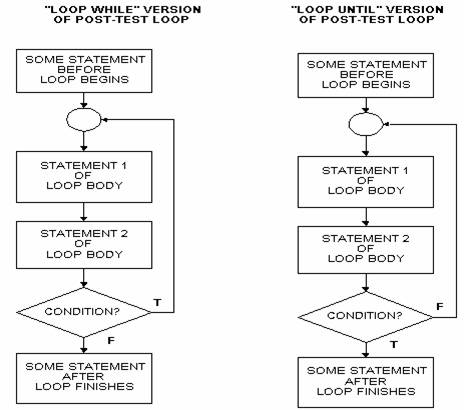The Do/Loop While (Loop Until) Loop

The general format for a post-test loop in VB is:

Do

<list of statements>

Loop {While | Until} <condition>

As an example of a post-test loop, the following is a code segment containing a Do/Loop construct that allows a user three tries to get his password right:

Dim strUserTry   As String

Dim intNumTries  As Integer

intNumTries = 0

Do

intNumTries = intNumTries + 1

Loop Until strUserTry = strPassWord Or intNumTries = 3

### Exiting a Loop Early

Occasionally, it may be necessary or more efficient to exit a loop early (i.e., before the loop termination decision is reached) due to some other condition that becomes true as the loop is processing. For this situation the Exit Do or Exit For statement can be used.

Example: Searching for a test value in an array – look at each element of the array 1 by 1, exiting when a match is found.

' variable declarations (whether some of these are declared at the module level

' or in a local sub would depend on the application) ...

Dim astrNames(0 To 9) As String

Dim strTestName       As String

Dim blnFoundIt        As Boolean

Dim intX              As Integer

' At some point in the program, after the array has been loaded and we have

' a test name to look for, the search logic might look like this:

blnFoundIt = False

For intX = 0 To 9

If strTestName = astrNames(intX) Then

blnFoundIt = True

Exit For

End If

Next

If blnFoundIt Then . . .

### Counting, Totaling and Averaging

Within loops, a variable that serves as a counter is frequently used to count how many times the loop has been repeated, thus reflecting how many items have been processed.  You saw that the For/Next loop has a built-in counter; but a limitation of the For/Next loop is that you must know in advance how many items will be processed in the loop.  With a Do While or Do Until loop, you typically do not know in advance how many items will be processed. With Do While or Do Until, your counter variable is typically initialized to zero or one, and you increment your counter variable with an assignment statement that has the form:

Counter = Counter + 1

When the loop exits, the counter variable contains a number that reflects the number of times the loop was executed.

Another type of variable frequently used within loops is a total or accumulator variable.  An accumulator variable is typically initialized to zero and then has an amount added to it each time that the loop repeats.  The amount that is added to the accumulator variable will generally be from an item that was obtained directly from input, or from a variable derived from a calculation based on one or more input items.  You add to your accumulator variable with an assignment statement that has the form:

Total = Total + Value

When the loop exits, the accumulator variable contains a value that reflects the accumulated total derived from repeatedly adding into it on each pass through the loop.

If your application calls for an average, your loop must perform both counting and totaling.  When the loop exits, you can calculate the average by dividing the total by the count, for example:

Average = Total / Counter